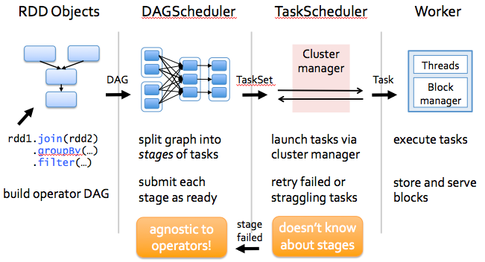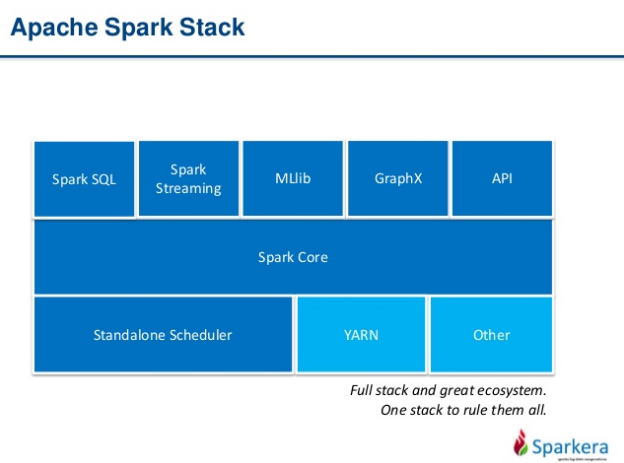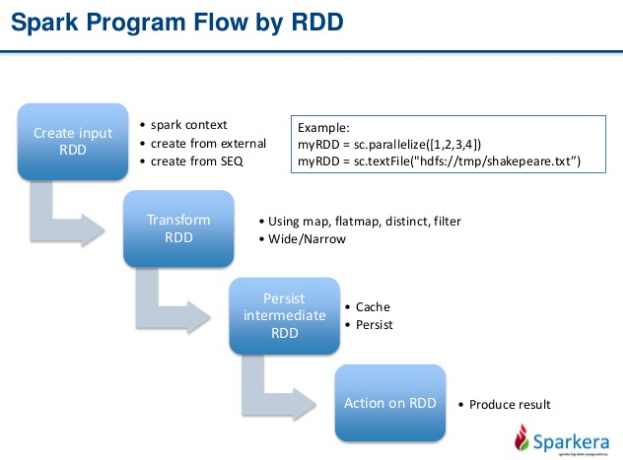#Spark Overview

Week 10 | Lesson 3.1

### LEARNING OBJECTIVES

After this lesson, you will be able to:

• describe what Spark is
• explain how Spark works
• identify how Spark is different from Hadoop
• tell some successful use cases of Spark
• be able to run simple queries in spark from the command line

### STUDENT PRE-WORK

Before this lesson, you should already be able to:

• perform map reduce jobs with Hadoop
• perform map reduce with Python MRJob

### INSTRUCTOR PREP

Before this lesson, instructors will need to:

• Read in / Review any dataset(s) & starter/solution code
• Generate a brief slide deck
• Prepare any specific materials
• Provide students with additional resources

### STARTER CODE

Code Along

This week requires additional prep in order to successfully run all lessons and labs provided:

• Note: Instructors will need to distribute individual URLs for the signup form. See linked instructions.

### LESSON GUIDE

TIMING TYPE TOPIC
5 min Opening Opening
20 min Introduction Intro to Spark
10 min Introduction2 Spark Stack and API
15 min Demo Demo: Spark Map Reduce
10 min Guided-practice Guided Practice: Spark Map Reduce
15 min Ind-practice Independent Practice: Explore the Spark Shell
10 min Conclusion Conclusion

## Opening (5 min)

Today will be dedicated to Spark. Spark has brought a revolution in Big Data in the past few years and it is thus important to introduce it and explain how it differes from Hadoop.

Check: can anyone recap what Map Reduce is and how it works?

It's a framework to solve problems that involve parallel calculation. It's composed of 2 steps: a mapper step in which multiple workers solve the same task on different parts of the dataset and a reducer phase where the results of each workers' work are combined to give a final result.

Check: what limitations have you encountered when processing data with Hadoop?

Mainly performance. It takes a long time to perform a map-reduce job, which makes it really hard to experiment and iterate.

## Intro to Spark (20 min)

Apache Spark is an open source cluster computing framework. Originally developed at the University of California, Berkeley's AMPLab, the Spark codebase was later donated to the Apache Software Foundation that has maintained it since. Spark provides an interface for programming entire clusters with implicit data parallelism and fault-tolerance.

Spark has gained traction over the past few years because of its superior performace with respect to Hadoop-MapReduce. In fact, Spark was developed in response to limitations in the MapReduce cluster computing paradigm. By now you should be familiar with MapReduce. In MapReduce data is read from disk and then a function is mapped across the data. Then the reducer will reduce the results of the map and finally store reduction results back to HDFS.

Spark relaxes the constraints of MR by doing the following:

• generalizes computation from Map/Reduce only graphs to arbitrary Directed Acyclic Graphs (DAGs)
• removes a lot of boilerplate code present in Hadoop
• allows to "tweak" parts that in Hadoop are not accessible, like for example the sort algorithm
• allows to load data in a cluster memory, speeding up I/O enormously

The two pillars on which Spark is based are RDDs and DAGs.

### Resilient Distributed Datasets (RDDs)

Apache Spark provides programmers with an application programming interface centered on a data structure called the resilient distributed dataset (RDD), a read-only multiset of data items distributed over a cluster of machines, that is maintained in a fault-tolerant way. Spark's RDDs function as a working set for distributed programs that offers a (deliberately) restricted form of distributed shared memory. In other words, Spark is keeping the data in memory, instead of on disk, thus making it easier to implement both iterative algorithms, that visit their dataset multiple times in a loop, and interactive/exploratory data analysis, i.e., the repeated database-style querying of data.

The latency of such applications (compared to Apache Hadoop, a popular MapReduce implementation) may be reduced by several orders of magnitude with this approach. Among the class of iterative algorithms are the training algorithms for machine learning systems, which formed the initial impetus for developing Apache Spark. In other words, with Spark we are able to perform Machine Learning at Big Data scale!

Apache Spark requires a cluster manager and a distributed storage system. For cluster management, Spark supports standalone (native Spark cluster), Hadoop YARN, or Apache Mesos. For distributed storage, Spark can interface with a wide variety, including Hadoop Distributed File System (HDFS), MapR File System (MapR-FS), Cassandra, OpenStack Swift, Amazon S3, Kudu, or a custom solution can be implemented. Spark also supports a pseudo-distributed local mode, usually used only for development or testing purposes, where distributed storage is not required and the local file system can be used instead; in such a scenario, Spark is run on a single machine with one executor per CPU core. This is how we will use it.

### Directed Acyclic Graph?

DAG (Directed Acyclic Graph) is a programming style for distributed systems - You can think of it as an alternative to Map Reduce. While MR has just two steps (map and reduce), DAG can have multiple levels that can form a tree structure. Say if you want to execute a SQL query, DAG is more flexible with more functions like map, filter, union etc. Each node in the graph represent a particular operation on the data. The graph is Directed, meaning the information only flows in one direction along the edges and it cannot flow backwards. This is makes the identification of inputs and outputs easy and unique.As we will see Spark is smart in how it performs the calculation along the DAG.Check: can you draw a DAG for Hadoop Map Reduce?

Answer: see picture in the assets/images folder

## Spark Stack and API (10 min)The Spark Core is the foundation of the overall project. It provides distributed task dispatching, scheduling, and basic I/O functionalities, exposed through an application programming interface (for Java, Python, Scala, and R) centered on the RDD abstraction.

Here are some of the operations offered by the Spark API:

• map
• filter
• groupby
• union
• sort
• join
• reduce
• count
• fold
• reduceByKey
• cogroup
• cross
• zip
• sample
• take
• ....

Spark is built in Scala, a language derived from Java, that has all the support for functional programing languages, but also has support for OOP languages. Spark builds computation by concatenating functions in the DAG.#### Spark Variables

Spark provides two forms of shared variables:

• broadcast variables: they reference read-only data that needs to be available on all nodes
• accumulators: they can be used to program reductions in an imperative style

#### Spark Operations

Spark provides two types of operations:

• Transformations: these are "lazy" operations that only return a result upon "collect"
• Actions: these are "non-lazy" operations that immediately return a result

Using lazy operations, we can build a computation graph that only gets executed when we collect the result. This allows Spark to optimize the requested calculation by optimizing the underlying DAG of operations.

Check: In pairs: each person chooses one of the operations listed above and looks how it works in the documentation. Then you have 2 minutes to explain each other what you have learned.

## Demo: Spark Map Reduce (15 min)

For the next part we will need our Virtual Machine. By now you should be familiar with how to start it and use it.

• Launch Virtualbox
• Start Bigdata VM
• open a terminal window
• connect with ssh [email protected]
• run bigdata_start.sh

Let's open a PySpark shell by typing: `pyspark`.You should also be able to see an active spark context here: http://10.211.55.101:4040/jobs/Let's load a text file and perform a few operations:

``````textFile = sc.textFile('file:///home/vagrant/data/project_gutenberg/pg11.txt')
``````

We have just created an RDD called `textFile`. As you know, RDDs have actions, which return values, and transformations, which return pointers to new RDDs. Let’s start with a few actions:

``````>>> textFile.count() # Number of items in this RDD
3735

>>> textFile.first() # First item in this RDD
u"Project Gutenberg's Alice's Adventures in Wonderland, by Lewis Carroll"
``````

Now let’s use a transformation. We will use the filter transformation to return a new RDD with a subset of the items in the file.

``````>>> linesWithAlice = textFile.filter(lambda line: "Alice" in line)
``````

Notice that the shell returned immediately, since this is a transformation which is lazy. If you type `linesWithAlice`, you should see:

``````PythonRDD at RDD at PythonRDD.scala:43
``````

We can chain together transformations and actions:

``````>>> textFile.filter(lambda line: "Alice" in line).count() # How many lines contain "Alice"?
396
``````

RDD actions and transformations can be used for more complex computations. Let’s say we want to find the line with the most words:

``````>>> textFile.map(lambda line: len(line.split())).reduce(lambda a, b: a if (a > b) else b)
18
``````

Let's stop here for a second and review the last line.

We started with the `textFile` RDD and we first applied the following map: `lambda line: len(line.split())`

Check: what does this function do?

Answer: it splits each line into words and counts how many words per line there are

the we chained to the result of the map the following reduce map: `lambda a, b: a if (a > b) else b`

Check what does this function do?

Answer: it takes two values and returns the biggest of the two

We used Python anonymous functions (lambdas), but we can also pass any top-level Python function we want. For example, we’ll define a max function to make this code easier to understand:

``````>>> def max(a, b):
...     if a > b:
...         return a
...     else:
...         return b
...

>>> textFile.map(lambda line: len(line.split())).reduce(max)
18
``````

## Guided Practice: Spark Map Reduce (10 min)

### Word count

Check: how did we implement the word count in Hadoop?

step 1: split into words step 2: map each word to a pair of (word, 1) step 3: reduce by key and sum the counts

Here is how you would implement it in Spark

``````>>> wordCounts = textFile.flatMap(lambda line: line.split()).map(lambda word: (word, 1)).reduceByKey(lambda a, b: a+b)
``````

Notice that this is a lazy operation, to collect the word counts in our shell, we can use the collect action:

``````>>> wordCounts.collect()
[(u"figure!'", 1), (u'four', 6), (u'hanging', 3), (u'ringlets', 1), (u"story!'", 2), (u'Foundation', 14), ...]
``````

To do this we have used 3 different Spark operations:

• map: Return a new distributed dataset formed by passing each element of the source through a function func
• flatMap: Similar to map, but each input item can be mapped to 0 or more output items. Using this we are given a single list of all the words in the file instead of a list of lists where each item is the list of words in a given line.
• reduceByKey: When called on a dataset of (K, V) pairs, returns a dataset of (K, V) pairs where the values for each key are aggregated using the given reduce function func, which must be of type (V,V) => V. Like in groupByKey, the number of reduce tasks is configurable through an optional second argument.

Check: Try modifying the code you have just written.

• Try sorting the words by count to find the most common words

### Caching

Spark also supports pulling data sets into a cluster-wide in-memory cache. This is very useful when data is accessed repeatedly, such as when querying a small “hot” dataset or when running an iterative algorithm like PageRank. As a simple example, let’s mark our `linesWithAlice` dataset to be cached:

``````>>> linesWithAlice.cache()
``````

Now try running `linesWithAlice.count()` twice. What happens?

Answer: the first time takes longer than the second time, because the result is cached

## Independent Practice: Explore the Spark Shell (15 min)

### Pi Estimation

Let's estimate the value of π by "throwing darts" at a circle. We pick random points in the unit square ((0, 0) to (1,1)) and see how many fall in the unit circle. The fraction should be π / 4. Try changing the value of NUM_SAMPLES and see what happens.

``````from random import random

def sample(p):
x, y = random(), random()
return 1 if x*x + y*y < 1 else 0

NUM_SAMPLES = 100000
count = sc.parallelize(xrange(0, NUM_SAMPLES)).map(sample).reduce(lambda a, b: a + b)
print "Pi is roughly %f" % (4.0 * count / NUM_SAMPLES)
``````

Pi is roughly 3.137640

Check: What's going on? Why did we just do that?

Answer: We parallelized the generation of NUM_SAMPLES random numbers to estimate the value of Pi. Cool!

## Conclusion: (10 min)

We conclude by recap some of the core components of Spark:

• Spark Core: Contains the basic functionality of Spark; in particular the APIs that define RDDs and the operations and actions that can be undertaken upon them. The rest of Spark's libraries are built on top of the RDD and Spark Core.

• Spark SQL: Provides APIs for interacting with Spark via the Apache Hive variant of SQL called Hive Query Language (HiveQL). Every database table is represented as an RDD and Spark SQL queries are transformed into Spark operations. For those that are familiar with Hive and HiveQL, Spark can act as a drop-in replacement.

• Spark Streaming: Enables the processing and manipulation of live streams of data in real time. Many streaming data libraries (such as Apache Storm) exist for handling real-time data. Spark Streaming enables programs to leverage this data similar to how you would interact with a normal RDD as data is flowing in.

• MLlib: A library of common machine learning algorithms implemented as Spark operations on RDDs. This library contains scalable learning algorithms like classifications, regressions, etc. that require iterative operations across large data sets. The Mahout library, formerly the Big Data machine learning library of choice, will move to Spark for its implementations in the future.

• GraphX: A collection of algorithms and tools for manipulating graphs and performing parallel graph operations and computations. GraphX extends the RDD API to include operations for manipulating graphs, creating subgraphs, or accessing all vertices in a path.